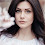## How to

When solving these problems, learning by doing is much better than learning by reading. I encourage to you read only as far in the solution as you need, then trying to solve the problem. If you get stuck, try reading a little further. And of course, let me know if you find a better solution!

## Thursday, February 20, 2014

### Implement Square Root (Part I)

Problem:  Write a function which returns the square root of an integer.  Return an integer which is the floor of the integer closest to the value.

int sqrtFlr(int x) {...

Solution:
Let's start off by doing an example:

Let's enter 75.  The square root of 75 is between 8 and 9.  Therefore, we would return the floor, or 8.

Now, let's think of how we determined the answer was 8.  Effectively, we knew squares until we found one that was just below (64) and just above (81) of 75.  Then, we returned the lesser.  We could imagine turning this into a relatively straight forward implementation of the problem, where we would try every number, until we found one that was just greater, and the return one below.

If we thing of this as an algorithm:
- Start at 1
- Iterate through numbers, squaring each
- Stop when you are greater than your number
- Return one less than the number you squared.

Now, if we code this, we get:

int sqrtFlr(int x) {

int i = 0;

while((i * i) < x) {
i++;
}

return i-1;
}

Now, let's try an example, 80.  We then start with i at 0, and end the while loop when i = 9.  And then, we correctly return 8.

Now, let's try some other cases.  Let's consider when x is itself a perfect square, say 36.  In this case we would stop the while lop when i = 6, as 36 is not less than 36.  So, this is a bug.  We would need to make the correct state i <= 6 to catch this case.

Also, let's imagine negative numbers.  As negative numbers require the imaginary number, i, we can just return -1 (or throw an error).

Also, let's think of 0.  0 is in fact a perfect square, so this will work if we change the < than sign to <=.

Ok, making these changes we get:

int sqrtFlr(int x) {

int i = 0;
if (x < 0) return -1; /* error case */

while((i * i) <= x) {
i++;
}

return i-1;
}

Let's consider the efficiency of this algorithm.  We go through every integer up to the square root ceiling (or one past for a perfect square), so this is an O(sqrt(n)) algorithm.

This is efficient, by O(sqrt(n)) is still quite big if x is big.  Consider that the sqrt of a massive number, like 2^256, is 2^128.

We can do much better.  That's next week's problem.

/* Please send me any bugs or optimizations.*/

1.thank you so much for sharing this informative blog . you can go
2.3.4.